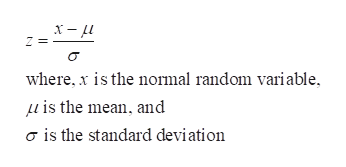# Assume Z is a random variable with a standard normal distribution and c is a positive number. If P(Z>c)=0.1 then P(−c<Z<c)=0.8.True or False

Question
43 views
Assume Z is a random variable with a standard normal distribution and c is a positive number. If P(Z>c)=0.1 then P(−c<Z<c)=0.8.

True or False

check_circle

Step 1

Standard normal distribution:

The standard normal distribution is a special case of normal distribution, in which the mean of the distribution ...help_outlineImage Transcriptionclosewhere, x is the normal random variable is the mean, and o is the standard deviation fullscreen

### Want to see the full answer?

See Solution

#### Want to see this answer and more?

Solutions are written by subject experts who are available 24/7. Questions are typically answered within 1 hour.*

See Solution
*Response times may vary by subject and question.
Tagged in

### Hypothesis Testing